# Go Math Grade 8 Answer Key Chapter 10 Transformations and Similarity

Be the first person to grab Go Math Grade 8 Answer Key Chapter 10 Transformations and Similarity to score good marks in the exam. Many students have loved the way of explanation given on Go Math Grade 8 Answer Key on our website. If you want to learn the best way of solving maths, students can immediately start their practice with the help of the Go Math Grade 8 Chapter 10 Transformations and Similarity Answer Key.

## Go Math Grade 8 Chapter 10 Transformations and Similarity Answer Key

The best learning comes with the best learning guide. So, to have the best online maths practice, follow the questions, answers, and explanations available on Go Math Grade 8 Answer Key Chapter 10 Transformations and Similarity. Download Go Math Grade 8 Chapter 10 Transformations and Similarity Answer Key PDF and begin your practice. The students can easily learn maths with the Go Math Grade 8 Solution Key.

Lesson 1: Properties of Dilations

Lesson 2: Algebraic Representations of Dilations

Lesson 3: Similar Figures

Model Quiz

Mixed Review

### Guided Practice – Properties of Dilations – Page No. 318

Use triangles ABC and A′B′C ′ for 1–5.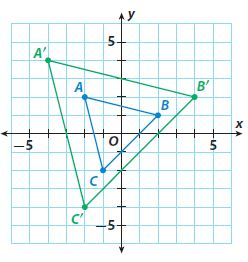Question 1.
For each pair of corresponding vertices, find the ratio of the x-coordinates and the ratio of the y-coordinates.
ratio of x-coordinates = _______
ratio of y-coordinates = _______
ratio of x-coordinates = ____________
ratio of y-coordinates = ____________

ratio of x-coordinates = 2
ratio of y-coordinates = 2

Explanation:
A’ = (-4, 4), A = (-2, 2);
ratio of x-coordinates = -4/-2 = 2
ratio of y-coordinates = 4/2 = 2
B’ = (4, 2), B = (2, 1);
ratio of x-coordinates = 4/2 = 2
ratio of y-coordinates = 2/1 = 2
C’ = (-2, -4), C = (-1, -2);
ratio of x-coordinates = -2/-1 = 2
ratio of y-coordinates = -4/-2 = 2

Question 2.
I know that triangle A′B′C ′ is a dilation of triangle ABC because the ratios of the corresponding x-coordinates are _______ and the ratios of the corresponding y-coordinates are _______.
Type below:
_____________

I know that triangle A′B′C ′ is a dilation of triangle ABC because the ratios of the corresponding x-coordinates are equal and the ratios of the corresponding y-coordinates are equal.

Question 3.
The ratio of the lengths of the corresponding sides of triangle A′B′C ′ and triangle ABC equals _______.
________

The ratio of the lengths of the corresponding sides of triangle A′B′C ′ and triangle ABC equals 2.

Properties of Dilations Answer Key Question 4.
The corresponding angles of triangle ABC and triangle A′B′C ′ are _______.
Type below:
_____________

The corresponding angles of triangle ABC and triangle A′B′C ′ are congruent.

Question 5.
The scale factor of the dilation is _______.
________

The scale factor of the dilation is 2.

ESSENTIAL QUESTION CHECK-IN

Question 6.
How can you find the scale factor of a dilation?
Type below:
_____________

Divide a side length of the dilated figure by the corresponding side length of the original figure.

### 10.1 Independent Practice – Properties of Dilations – Page No. 319

For 7–11, tell whether one figure is a dilation of the other or not. Explain your reasoning.

Question 7.
Quadrilateral MNPQ has side lengths of 15 mm, 24 mm, 21 mm, and 18 mm. Quadrilateral M′N′P′Q′ has side lengths of 5 mm, 8 mm, 7 mm, and 4 mm.
_____________

MNPQ is not a dilation of M′N′P′Q′

Explanation:
15/5 = 3 mm
24/8 = 3 mm
21/7 = 3 mm
18/4 = 4.5 mm
The ratios of the lengths of the corresponding sides are not equal.
Therefore, MNPQ is not a dilation of M′N′P′Q′

Question 8.
Triangle RST has angles measuring 38° and 75°. Triangle R′S′T ′ has angles measuring 67° and 38°. The sides are proportional.
_____________

Yes

Explanation:
Both Triangle S have Angle S of measures 38°, 67° and 75°. So, the corresponding ∠S are congruent.

Question 9.
Two triangles, Triangle 1 and Triangle 2, are similar.
_____________

Yes

Explanation:
a dilation produces an image similar to the original figure

Question 10.
Quadrilateral MNPQ is the same shape but a different size than quadrilateral M′N′P′Q.
_____________

Yes

Explanation:
The figures are similar is they are the same shape but different size SO one is a dilation of the other

Question 11.
On a coordinate plane, triangle UVW has coordinates U(20, −12), V(8, 6), and W(−24, -4). Triangle U′V′W′ has coordinates U′(15, −9), V′(6, 4.5), and W′(−18, -3).
_____________

Yes

Explanation:
Each coordinate of Triangle U′V′W′ is 3/4 times the corresponding coordinate of Triangle UVW.
So, the scale factor of the dilation is 3/4.

Complete the table by writing “same” or “changed” to compare the image with the original figure in the given transformation.

Question 12.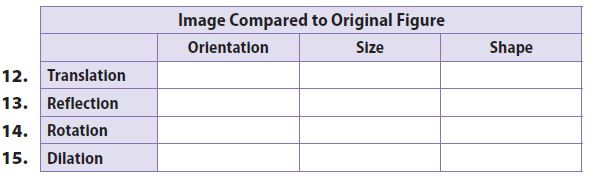Type below:
_____________Question 16.
Describe the image of a dilation with a scale factor of 1.
_____________

The image is congruent to the original figure

### Properties of Dilations – Page No. 320

Identify the scale factor used in each dilation.

Question 17.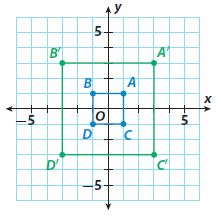________

3

Explanation:
A’B’/AB = 6/2 = 3
B’D’/BD = 6/2 = 3
scale factor = 3

Question 18.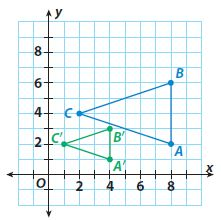$$\frac{□}{□}$$

$$\frac{1}{2}$$

Explanation:
A’B’/AB = 2/4 = 1/2
scale factor = 1/2

FOCUS ON HIGHER ORDER THINKING

Question 19.
Critical Thinking Explain how you can find the center of dilation of a triangle and its dilation.
Type below:
_____________

If you draw a line connecting each pair of corresponding vertices, the lines will intersect at the center of dilation

Dilations and Similarity Answer Key Question 20.
Make a Conjecture
a. A square on the coordinate plane has vertices at (−2, 2), (2, 2), (2, −2), and (−2, −2). A dilation of the square has vertices at (−4, 4), (4, 4), (4, −4), and (−4, −4). Find the scale factor and the perimeter of each square.
Scale factor: _________
Original perimeter: _________
Image perimeter: _________

Scale factor: 2
Original perimeter: 16
Image perimeter: 32

Explanation:
-4/-2 =2; 4/2 = 2
Scale factor = 2
perimeter of the original square = 4 + 4 + 4 + 4 = 16
perimeter of the image = 8 + 8 + 8 + 8 = 32

Question 20.
b. A square on the coordinate plane has vertices at (−3, 3), (3, 3), (3, −3), and (−3, −3). A dilation of the square has vertices at (−6, 6), (6, 6), (6, −6), and (−6, −6). Find the scale factor and the perimeter of each square.
Scale factor: _________
Original perimeter: _________
Image perimeter: _________

Scale factor: 2
Original perimeter: 24
Image perimeter: 48

Explanation:
-6/-3 =2; 6/3 = 2
Scale factor = 2
perimeter of the original square = 6 + 6 + 6 + 6 = 24
perimeter of the image = 12 + 12 + 12 + 12 = 48

Question 20.
c. Make a conjecture about the relationship of the scale factor to the perimeter of a square and its image.
Type below:
_____________

The perimeter of the image is the perimeter of the original figure times the scale factor

### Guided Practice – Algebraic Representations of Dilations – Page No. 324

Question 1.
The grid shows a diamond-shaped preimage. Write the coordinates of the vertices of the preimage in the first column of the table. Then apply the dilation (x, y) → ($$\frac{3}{2}$$x, $$\frac{3}{2}$$y) and write the coordinates of the vertices of the image in the second column. Sketch the image of the figure after the dilation.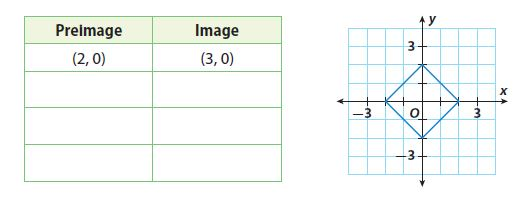Type below:
_____________Graph the image of each figure after a dilation with the origin as its center and the given scale factor. Then write an algebraic rule to describe the dilation.

Question 2.
scale factor of 1.5Type below:
_____________

(x, y) -> (1.5x, 1.5y)

Explanation:
After dilation
F’ (3, 3)
G’ (7.5, 3)
H’ (7.5, 6)
I’ (3, 6)
algebraic rule: (x, y) -> (1.5x, 1.5y)

Algebraic Representations of Dilations Lesson 10.2 Answers Question 3.
scale factor of $$\frac{1}{3}$$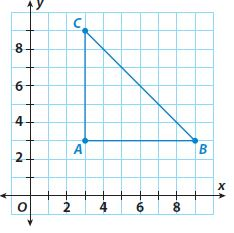Type below:
_____________

(x, y) -> (1/3x, 1/3y)

Explanation:
After dilation
A’ (3, 3)
B’ (7.5, 3)
C’ (7.5, 6)
algebraic rule: (x, y) -> (1/3x, 1/3y)

ESSENTIAL QUESTION CHECK-IN

Question 4.
A dilation of (x, y) → (kx, ky) when 0 < k < 1 has what effect on the figure? What is the effect on the figure when k > 1?
Type below:
_____________

When k is between 0 and 1, the dilation is a reduction by the scale factor k.
When k is greater than 1, the dilation is an enlargement by the scale factor k.

### 10.2 Independent Practice – Algebraic Representations of Dilations – Page No. 325

Question 5.
The blue square is the preimage. Write two algebraic representations, one for the dilation to the green square and one for the dilation to the purple square.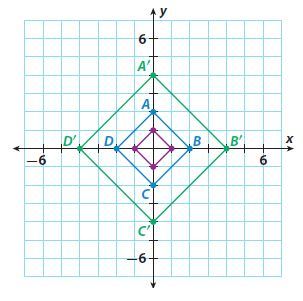Type below:
_____________

Green square -> (x, y) -> (2x, 2y)
Purple square -> (x, y) -> (1/2x, 1/2y)

Question 6.
Critical Thinking A triangle has vertices A(-5, -4), B(2, 6), and C(4, -3). The center of dilation is the origin and (x, y) → (3x, 3y). What are the vertices of the dilated image?
Type below:
_____________

A'(-15, -12)
B'(6, 18)
C'(12, -9)

Explanation:
A'((3.-15), (3.-12)) -> A'(-15, -12)
B'((3. 2), (3.6)) -> B'(6, 18)
C'((3. 4), (3.-3)) -> C'(12, -9)

Critical Thinking M′N′O′P′ has vertices at M′(3, 4), N′(6, 4), O′(6, 7), and P′(3, 7). The center of dilation is the origin. MNOP has vertices at M(4.5, 6), N(9, 6), O′(9, 10.5), and P′(4.5, 10.5). What is the algebraic representation of this dilation?
Type below:
_____________

(x, y) -> (2/3x, 2/3y)

Explanation:
M’N’/MN = 3/4.5 = 2/3
algebraic rule: (x, y) -> (2/3x, 2/3y)

Question 8.
Critical Thinking A dilation with center (0,0) and scale factor k is applied to a polygon. What dilation can you apply to the image to return it to the original preimage?
Type below:
_____________

A dilation with scale factor 1/k

Question 9.
Represent Real-World Problems The blueprints for a new house are scaled so that $$\frac{1}{4}$$ inch equals 1 foot. The blueprint is the preimage and the house is the dilated image. The blueprints are plotted on a coordinate plane.
a. What is the scale factor in terms of inches to inches?
Scale factor: ________

Scale factor: 48

Explanation:
scale factor = 48

Question 9.
b. One inch on the blueprint represents how many inches in the actual house? How many feet?
________ inches
________ feet

48 inches
4 feet

Explanation:
48 inches or 4 feet

Question 9.
c. Write the algebraic representation of the dilation from the blueprint to the house.
Type below:
_____________

(x, y) -> (48x, 48y)

Question 9.
d. A rectangular room has coordinates Q(2, 2), R(7, 2), S(7, 5), and T(2, 5) on the blueprint. The homeowner wants this room to be 25% larger. What are the coordinates of the new room?
Type below:
_____________

Q'(2.5, 2.5),
R'(8.75, 2.5),
S'(8.75, 6.25),
T'(2.5, 6.25)

Question 9.
e. What are the dimensions of the new room, in inches, on the blueprint? What will the dimensions of the new room be, in feet, in the new house?
Type below:
_____________

Blueprint dimensions: 6.25 in. by 3.75 in.
House dimensions: 25ft by 15ft

### Algebraic Representations of Dilations – Page No. 326

Question 10.
Write the algebraic representation of the dilation shown.Type below:
_____________

(x, y) -> (1/4x, 1/4y)

Explanation:
algebraic rule of the dilation: (x, y) -> (1/4x, 1/4y)

FOCUS ON HIGHER ORDER THINKING

Question 11.
Critique Reasoning The set for a school play needs a replica of a historic building painted on a backdrop that is 20 feet long and 16 feet high. The actual building measures 400 feet long and 320 feet high. A stage crewmember writes (x, y) → ($$\frac{1}{12}$$x, $$\frac{1}{12}$$y) to represent the dilation. Is the crewmember’s calculation correct if the painted replica is to cover the entire backdrop? Explain.
_____________

The stage crewmember’s calculation is incorrect.
The backdrop scale factor is 1/20, not 1/12

10.2 Independent Practice Answer Key Question 12.
Communicate Mathematical Ideas Explain what each of these algebraic transformations does to a figure.
a. (x, y) → (y, -x)
Type below:
_____________

(x, y) → (y, -x)
90º clockwise rotation

Question 12.
b. (x, y) → (-x, -y)
Type below:
_____________

(x, y) → (-x, -y)
180º rotation

Question 12.
c. (x, y) → (x, 2y)
Type below:
_____________

(x, y) → (x, 2y)
vertically stretches by a factor of 2

Question 12.
d. (x, y) → ($$\frac{2}{3}$$x, y)
Type below:
_____________

(x, y) → ($$\frac{2}{3}$$x, y)
horizontally shrinks by a factor of 2/3

Question 12.
e. (x, y) → (0.5x, 1.5y)
Type below:
_____________

(x, y) → (0.5x, 1.5y)
horizontally shrinks by a factor of 0.5 and vertically stretches by a factor of 1.5

Question 13.
Communicate Mathematical Ideas Triangle ABC has coordinates A(1, 5), B(-2, 1), and C(-2, 4). Sketch triangle ABC and A′B′C′ for the dilation (x, y) → (-2x, -2y). What is the effect of a negative scale factor?
Type below:
_____________

The figure is dilated by a factor of 2, but the orientation of the figure in the coordinate plane is rotated 180°

### Guided Practice – Similar Figures – Page No. 330

Question 1.
Apply the indicated sequence of transformations to the square. Apply each transformation to the image of the previous transformation. Label each image with the
letter of the transformation applied.A: (x, y) → (-x, y)
B: Rotate the square 180° around the origin.
C: (x, y) → (x – 5, y – 6)
D: (x, y) → ($$\frac{1}{2}$$x, $$\frac{1}{2}$$y)
Type below:
_____________

Explanation:
A: (x, y) → (-x, y)
coordinates for A
(-7, -8)
(-7, -4)
(-3, -4)
(-3, -8)
B: Rotate the square 180° around the origin.
coordinates for B
(3, 4)
(3, 8)
(7, 8)
(7, 4)
C: (x, y) → (x – 5, y – 6)
coordinates for C
(-2, -2)
(-2, 2)
(2, 2)
(2, -2)
D: (x, y) → ($$\frac{1}{2}$$x, $$\frac{1}{2}$$y)
coordinates for D
(-1, -1)
(-1, 1)
(1, 1)
(1, -1)

Identify a sequence of two transformations that will transform Figure A into the given figure.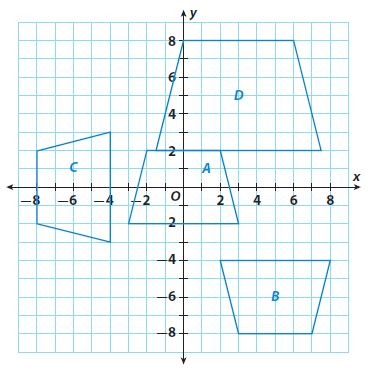Question 2.
figure B
Type below:
_____________

(x, y) -> (x, -y)
(x, y) -> (x +5, y-6)

Question 3.
figure C
Type below:
_____________

(x, y) -> (x, y+6)
rotate 90º counterclockwise

Question 4.
figure D
Type below:
_____________

(x, y) -> (1.5x, 1.5y)
(x, y) -> (x+3, y+5)

ESSENTIAL QUESTION CHECK-IN

Question 5.
If two figures are similar but not congruent, what do you know about the sequence of transformations used to create one from the other?
Type below:
_____________

At least one transformation must be a dilation with a scale factor other than 1

### 10.3 Independent Practice – Similar Figures – Page No. 331

Question 6.
A designer creates a drawing of a triangular sign on centimeter grid paper for a new business. The drawing has sides measuring 6 cm, 8 cm, and 10 cm, and angles measuring 37°, 53°, and 90°. To create the actual sign shown, the drawing must be dilated using a scale factor of 40.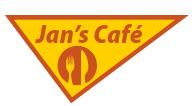a. Find the lengths of the sides of the actual sign.
Type below:
_____________

240 cm, 320 cm, and 400 cm

Explanation:
6cm × 40 = 240cm
8cm × 40 = 320cm
10cm × 40 = 400cm
The lengths are 240 cm, 320 cm, and 400 cm

Question 6.
b. Find the angle measures of the actual sign.
Type below:
_____________

The angle measures are the same
37º, 53º, and 90º

Question 6.
c. The drawing has the hypotenuse on the bottom. The business owner would like it on the top. Describe two transformations that will do this.
Type below:
_____________

Reflect the drawing over the x-axis
Rotate the drawing 180º around the origin.

Question 6.
d. The shorter leg of the drawing is currently on the left. The business owner wants it to remain on the left after the hypotenuse goes to the top. Which transformation in part c will accomplish this?
Type below:
_____________

Reflecting over the x-axis

In Exercises 7–10, the transformation of a figure into its image is described. Describe the transformations that will transform the image back into the original figure. Then write them algebraically.

Question 7.
The figure is reflected across the x-axis and dilated by a scale factor of 3.
Type below:
_____________

Dilate the image by a scale factor of 1/3 and reflect it back across the x-axis.
(x, y) -> (1/3x, 1/3y)

The figure is dilated by a scale factor of 0.5 and translated 6 units left and 3 units up.
Type below:
_____________

Translate the image 3 units down and 6 units right and dilate it by a factor of 2
(x, y) -> (x+6, y-3)
(x, y) -> (2x, 2y)

Question 9.
The figure is dilated by a scale factor of 5 and rotated 90° clockwise.
Type below:
_____________

Rotate the image 90 counterclockwise and dilate it by a factor of 1/5.
(x, y) -> (-y, x)
(x, y) -> (1/5x, 1/5y)

### Similar Figures – Page No. 332

Question 10.
The figure is reflected across the y-axis and dilated by a scale factor of 4.
Type below:
_____________

Dilate the image by a factor of 1/4 and reflect it back across the y-axis.
(x, y) -> (1/4x, 1/4y)
(x, y) -> (-x, y)

FOCUS ON HIGHER ORDER THINKING

Question 11.
Draw Conclusions A figure undergoes a sequence of transformations that include dilations. The figure and its final image are congruent. Explain how this can happen.
Type below:
_____________

There must be an even number of dilations and for each dilation applied to the figure, a dilation that has the opposite effect must be applied as well.

Question 12.
Multistep As with geometric figures, graphs can be transformed through translations, reflections, rotations, and dilations. Describe how the graph of y = x shown at the right is changed through each of the following transformations.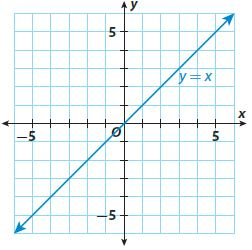a. a dilation by a scale factor of 4
Type below:
_____________

original coordinates
(0, -6)
(0, -4)
(4, 0)
(0, 4)
(-4, 4)
(-4, 2)
(-2, 0)
(-4, -2)

Question 12.
b. a translation down 3 units
Type below:
_____________

coordinates for A
(0, -4)
(0, -3)
(2, -1)
(0, 1)
(-2, 1)
(-2, 0)
(-1, -1)
(-2, -2)

Question 12.
c. a reflection across the y-axis
Type below:
_____________

coordinates for B
(-4, 3)
(-4, 2)
(-2, 0)
(-4, -2)
(-6, -2)
(-6, -1)
(-5, 0)
(-6, 1)

Question 13.
Justify Reasoning The graph of the line y = x is dilated by a scale factor of 3 and then translated up 5 units. Is this the same as translating the graph up 5 units and then dilating by a scale factor of 3? Explain.
Type below:
_____________

No; the dilation is not the same reversed

Explanation:
The position of the sketch from 12A will be 1/2 unit above the sketch obtained when the translation occurs first

### Ready to Go On? – Model Quiz – Page No. 333

10.1 Properties of Dilations

Determine whether one figure is a dilation of the other. Justify your answer.

Question 1.
Triangle XYZ has angles measuring 54° and 29°. Triangle X′Y′Z′ has angles measuring 29° and 92°.
_____________

No; one figure is not a dilation of the other

Explanation:
The triangles have only one pair of congruent angles

Quadrilateral DEFG has sides measuring 16 m, 28 m, 24 m, and 20 m. Quadrilateral D′E′F′G′ has sides measuring 20 m, 35 m, 30 m, and 25 m.
_____________

Explanation:
each side of the second figure is 1.25 times the corresponding side of the original figure.

10.2 Algebraic Representations of Dilations

Dilate each figure with the origin as the center of dilation.

Question 3.
(x, y) → (0.8x, 0.8y)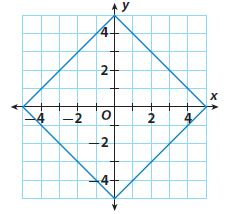Type below:
_____________

Coordinates after dilation
(0, -4)
(4, 0)
(0, 4)
(-4, 0)

Question 4.
(x, y) → (2.5x, 2.5y)Type below:
_____________

Coordinates after dilation
(-2.5, 2.5)
(5, 5)
(5, -3)

10.3 Similar Figures

Question 5.
Describe what happens to a figure when the given sequence of transformations is applied to it: (x, y) → (-x, y); (x, y) → (0.5x, 0.5y); (x, y) → (x – 2, y + 2)
Type below:
_____________

After the sequencing of transformations, reflection over the y-axis.
dilation with a scale factor of 0.5
translation 2 units left and 2 units up

ESSENTIAL QUESTION

Question 6.
How can you use dilations to solve real-world problems?
Type below:
_____________

You can use dilations when drawing or designing

### Selected Response – Mixed Review – Page No. 334

Question 1.
A rectangle has vertices (6, 4), (2, 4), (6, –2), and (2, –2). What are the coordinates of the vertices of the image after a dilation with the origin as its center and a scale factor of 1.5?
Options:
a. (9, 6), (3, 6), (9, –3), (3, –3)
b. (3, 2), (1, 2), (3, –1), (1, –1)
c. (12, 8), (4, 8), (12, –4), (4, –4)
d. (15, 10), (5, 10), (15, –5), (5, –5)

a. (9, 6), (3, 6), (9, –3), (3, –3)

Explanation:
(9 -3)/(6 -2) = 6/4 = 1.5

Question 2.
Which represents the dilation shown where the black figure is the preimage?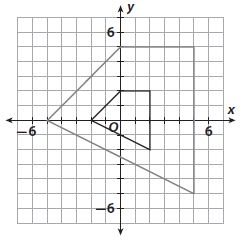Options:
a. (x, y) -> (1.5x, 1.5y)
b. (x, y) -> (2.5x, 2.5y)
c. (x, y) -> (3x, 3y)
d. (x, y) -> (6x, 6y)

b. (x, y) -> (2.5x, 2.5y)

Explanation:
5/2 = 2.5
10/4 = 2.5
(x, y) -> (2.5x, 2.5y)

Question 3.
Identify the sequence of transformations that will reflect a figure over the x-axis and then dilate it by a scale factor of 3.
Options:
a. (x, y) -> (-x, y); (x, y) -> (3x, 3y)
b. (x, y) -> (-x, y); (x, y) -> (x, 3y)
c. (x, y) -> (x, -y); (x, y) -> (3x, y)
d. (x, y) -> (x, -y); (x, y) -> (3x, 3y)

d. (x, y) -> (x, -y); (x, y) -> (3x, 3y)

Explanation:
Reflection over x-axis (x, y) -> (x, -y)
dilation by scale factor of 3 (x, y) -> (3x, 3y)
(x, y) -> (x, -y); (x, y) -> (3x, 3y)

Question 4.
Solve −a + 7 = 2a − 8.
Options:
a. a = -3
b. a = −$$\frac{1}{3}$$
c. a = 5
d. a = 15

c. a = 5

Explanation:
-a + 7 = 2a – 8
2a + a = 8 + 7
3a = 15
a = 15/3
a = 5

Which equation does not represent a line with an x-intercept of 3?
Options:
a. y = −2x + 6
b. y = −$$\frac{1}{3}$$x + 1
c. y = $$\frac{2}{3}$$x − 2
d. y = 3x − 1

d. y = 3x − 1

Explanation:
y = -2x + 6
0 = -2x + 6
2x = 6
x = 3
y = -1/3 . x + 1
0 = -1/3 . x + 1
1/3x = 1
x = 3
y = 2/3 . x – 2
0 = 2/3 . x – 2
2/3x = 2
x = 2 . 3/2
x = 3
y = 3x – 1
0 = 3x – 1
3x = 1
x = 1/3

The square is dilated under the dilation (x, y) → (0.25x, 0.25y).a. Graph the image. What are the coordinates?
Type below:
_____________

After dilation:
(-1, 1)
(1, 1)
(1, -1)
(-1, -1)

Question 6.
b. What is the length of a side of the image?
______ units

2 units

Explanation:
The length is 2 units

Question 6.
c. What are the perimeter and area of the preimage?
Perimeter = ________ units
Area = ________ square units

Perimeter = 32 units
Area = 64 square units

Explanation:
Perimeter = 2l + 2w = 2(8) + 2(8) = 32
area = l.w = 8 .8 = 64

Question 6.
d. What are the perimeter and area of the image?
Perimeter = ________ units
Area = ________ square units